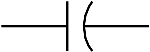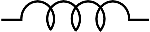Science >> Physics for Kids

# Physics for Kids

## Resistors, Capacitors, and Inductors

The three basic elements used in electronic circuits are the resistor, capacitor, and inductor. They each play an important role in how an electronic circuit behaves. They also have their own standard symbols and units of measurement.

Resistors

A resistor represents a given amount of resistance in a circuit. Resistance is a measure of how the flow of electric current is opposed or "resisted." It is defined by Ohm's law which says the resistance equals the voltage divided by the current.

Resistance = voltage/current
or
R = V/I

Resistance is measured in Ohms. The Ohm is often represented by the omega symbol: Ω.

The symbol for resistance is a zigzag line as shown below. The letter "R" is used in equations.Resistor Symbol

Capacitors

A capacitor represents the amount of capacitance in a circuit. The capacitance is the ability of a component to store an electrical charge. You can think of it as the "capacity" to store a charge. The capacitance is defined by the equation

C = q/V
where q is the charge in coulombs and V is the voltage.

In a DC circuit, a capacitor becomes an open circuit blocking any DC current from passing the capacitor. Only AC current will pass through a capacitor.

The symbol for capacitance is two parallel lines. Sometimes one of the lines is curved as shown below. The letter "C" is used in equations.Capacitor Symbol

Inductors

An inductor represents the amount of inductance in a circuit. The inductance is the ability of a component to generate electromotive force due to a change in the flow of current. A simple inductor is made by looping a wire into a coil. Inductors are used in electronic circuits to reduce or oppose the change in electric current.

In a DC circuit, an inductor looks like a wire. It has no affect when the current is constant. Inductance only has an effect when the current is changing as in an AC circuit.

Inductance is measured in Henrys.

The symbol for inductance is a series of coils as shown below. The letter "L" is used in equations.Inductor Symbol

Interesting Facts about Resistors, Capacitors, and Inductors
• The resistance of a material is the opposite or the inverse of the conductivity.
• The Ohm is named after German physicist Georg Ohm.
• The Henry is named after American scientist Joseph Henry.
• Combinations of capacitors, inductors, and resistors are used to build passive filters that will only allow electronic signals of certain frequencies to pass through.
Activities

More Electricity Subjects

Science >> Physics for Kids